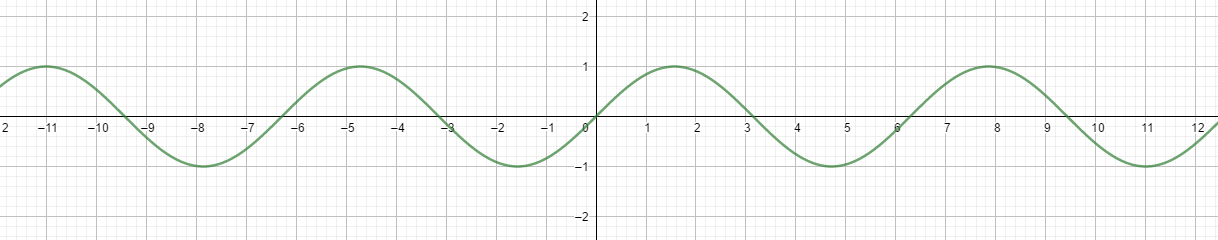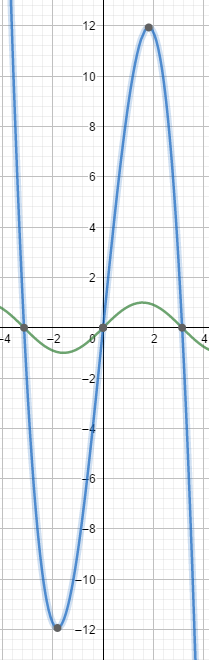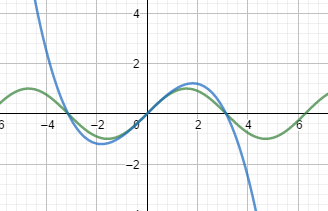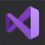# Why $\frac{1}{1^2} + \frac{1}{2^2} + \frac{1}{3^2} + \cdots = \frac{\pi^2}{6}$

In the previous note that I've written I said $\sin x = \frac{x}{1!} - \frac{x^3}{3!} + \frac{x^5}{5!} - \frac{x^7}{7!} + \cdots$ This can come in handy today! $\newline$ We know the function image of $\sin x$ is:First we can say $\sin x \approx (\pi + x)x(\pi -x)$ because when $x = -\pi$ or $0$ or $\pi$ the $(\pi +x)x(\pi -x) = 0$ and $\sin x = 0$. But let we see the function image (the blue line):This fits too badly! $(\sin x)' = \cos x$ and when $x = 0$ then $(\sin x)' = 1$. Can we let $[C_1 \times (\pi +x)x(\pi -x)]' = 1$ when $x = 0$? $\newline$ Let $[C_1 \times (\pi +x)x(\pi -x)]' = 1$ which $C_1$ is a constant. So $C_1 \times [(\pi +x)x(\pi -x)]' = 1$ when $x = 0$. After expansion, derivation, only "$\pi ^2$" is left, and the others are all 0 because they contain x (ie 0). So $C_1 \times \pi ^2 = 1$ $C_1 = \frac{1}{\pi ^2}$ $\frac{1}{\pi ^2} (\pi + x)x(\pi -x) = (1+\frac{x}{\pi})x(1-\frac{x}{\pi})$ Then it will become like this:It's great! $\newline$The same, $\sin x \approx (2\pi +x)(1+\frac{x}{\pi})x(1-\frac{x}{\pi})(2\pi -x)$ $C_2 \times [(2\pi +x)(1+\frac{x}{\pi})x(1-\frac{x}{\pi})(2\pi -x)]' = 1$ only "$(2\pi)^2$" is left, and the others are all 0 because they contain x (ie 0). So $C_2 = \frac{1}{(2\pi)^2}$ $\frac{1}{(2\pi)^2}(2\pi +x)(1+\frac{x}{\pi})x(1-\frac{x}{\pi})(2\pi -x) = (1 +\frac{x}{2\pi})(1+\frac{x}{\pi})x(1-\frac{x}{\pi})(1 -\frac{x}{(2\pi)^2})$ And so on: $C_3 = \frac{1}{(3\pi)^2}, C_4=\frac{1}{(4\pi)^2}, C_n=\frac{1}{(n\pi)^2 }\cdots$ Therefore $\sin x = \cdots (1 +\frac{x}{2\pi})(1+\frac{x}{\pi})x(1-\frac{x}{\pi})(1 -\frac{x}{(2\pi)^2}) \cdots$ We also know $(a+b)(a-b)=a^2-b^2$. Then $\sin x = x(1-\frac{x^2}{\pi^2})(1-\frac{x^2}{(2\pi)^2})(1-\frac{x^2}{(3\pi)^2}) \cdots$ and $\sin x$ also is $\frac{x}{1!} - \frac{x^3}{3!} + \frac{x^5}{5!} - \frac{x^7}{7!} + \cdots$ So $\frac{x}{1!} - \frac{x^3}{3!} + \frac{x^5}{5!} - \frac{x^7}{7!} + \cdots = x(1-\frac{x^2}{\pi^2})(1-\frac{x^2}{(2\pi)^2})(1-\frac{x^2}{(3\pi)^2}) \cdots$ Therefore, the split items with $x^3$ items on the left and right are equal. In the left of the formula, it has $-x^3 \frac{1}{3!}$ and in the right of the formula -- how many $x^3$ does it have? We must have $x^3$, remove the first $x$, it takes a $\frac{x^2}{\cdots}$ to form $x^3$. So we have: $x^3 \times (-\frac{1}{3!})=x(-\frac{x^2}{\pi ^2} - \frac{x^2}{(2\pi)^2} - \frac{x^2}{(3\pi)^2}-\cdots)$ $\frac{1}{3!} = \frac{1}{\pi^2} + \frac{1}{(2\pi)^2} + \frac{1}{(3\pi)^2} + \cdots$ $\frac{1}{6} = \frac{1}{\pi^2}(\frac{1}{1^2} + \frac{1}{2^2} + \frac{1}{(3\pi)^2} + \cdots)$ $\frac{\pi^2}{6} = \frac{1}{1^2} + \frac{1}{2^2} + \frac{1}{3^2} + \cdots$ Proven!Note by Raymond Fang
4 months, 2 weeks ago

This discussion board is a place to discuss our Daily Challenges and the math and science related to those challenges. Explanations are more than just a solution — they should explain the steps and thinking strategies that you used to obtain the solution. Comments should further the discussion of math and science.

When posting on Brilliant:

• Use the emojis to react to an explanation, whether you're congratulating a job well done , or just really confused .
• Ask specific questions about the challenge or the steps in somebody's explanation. Well-posed questions can add a lot to the discussion, but posting "I don't understand!" doesn't help anyone.
• Try to contribute something new to the discussion, whether it is an extension, generalization or other idea related to the challenge.
• Stay on topic — we're all here to learn more about math and science, not to hear about your favorite get-rich-quick scheme or current world events.

MarkdownAppears as
*italics* or _italics_ italics
**bold** or __bold__ bold
- bulleted- list
• bulleted
• list
1. numbered2. list
1. numbered
2. list
Note: you must add a full line of space before and after lists for them to show up correctly
paragraph 1paragraph 2

paragraph 1

paragraph 2

[example link](https://brilliant.org)example link
> This is a quote
This is a quote
    # I indented these lines
# 4 spaces, and now they show
# up as a code block.

print "hello world"
# I indented these lines
# 4 spaces, and now they show
# up as a code block.

print "hello world"
MathAppears as
Remember to wrap math in $$ ... $$ or $ ... $ to ensure proper formatting.
2 \times 3 $2 \times 3$
2^{34} $2^{34}$
a_{i-1} $a_{i-1}$
\frac{2}{3} $\frac{2}{3}$
\sqrt{2} $\sqrt{2}$
\sum_{i=1}^3 $\sum_{i=1}^3$
\sin \theta $\sin \theta$
\boxed{123} $\boxed{123}$

## Comments

Sort by:

Top Newest

Great! in this way you can also find the sum like $\zeta(4)=\sum_{n=1}^∞ \dfrac{1}{n^4}= \dfrac{π^4}{90}$ $\zeta(6)=\sum_{n=1}^∞ \dfrac{1}{n^6}= \dfrac{π^6}{945}$ $\zeta(8)=\sum_{n=1}^∞ \dfrac{1}{n^8}= \dfrac{π^8}{9450}$ $\zeta(10)=\sum_{n=1}^∞ \dfrac{1}{n^{10}}= \dfrac{π^{10}}{93555}$ For General $\zeta(2n)= \dfrac{(-1)^{n+1} \beta_{2n} (2π)^{2n}}{2(2n)!}$ Where $\beta_n$= $n^{th}$ Bernoulli Number

- 4 months, 2 weeks ago

Log in to reply

Great!

- 4 months, 2 weeks ago

Log in to reply

×

Problem Loading...

Note Loading...

Set Loading...